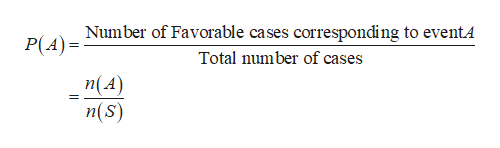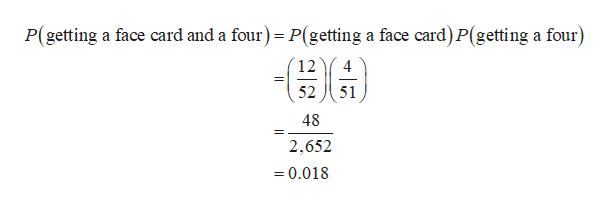# a. From a standard shuffled deck of cards, find the following probabilities:A draw of two cards without replacement that result in a face card then a fourb. The table below represents the results of gender prediction from an ultrasound Predicted MalePredicted FemaleBaby is Male7412Baby is Female1459Find the following probabilities:Correct PredictionPrediction is male given that the baby is male

Question

a. From a standard shuffled deck of cards, find the following probabilities:

1. A draw of two cards without replacement that result in a face card then a four

b. The table below represents the results of gender prediction from an ultrasound

 Predicted Male Predicted Female Baby is Male 74 12 Baby is Female 14 59

Find the following probabilities:

1. Correct Prediction
2. Prediction is male given that the baby is male
check_circle

Step 1

For any event A and the sample space S, the probability can be found as,help_outlineImage TranscriptioncloseNumber of Favorable cases corresponding to eventA P(A) Total number of cases n(A) n(s) fullscreen
Step 2

Conditional rule:

The formula for probability of A given B is:

Step 3

a. The probability of getting a face card and then a four:

There are 12 face cards and 4 fours’ in a deck of 52 cards. The two cards are drawn one after the other without replacement. The probability of getting a face card first from the ...help_outlineImage TranscriptioncloseP(getting a face card and a four) P(getting a face card) P(getting a four) 12 4 = 52 51 48 2,652 =0.018 fullscreen

### Want to see the full answer?

See Solution

#### Want to see this answer and more?

Solutions are written by subject experts who are available 24/7. Questions are typically answered within 1 hour.*

See Solution
*Response times may vary by subject and question.
Tagged in

### Other